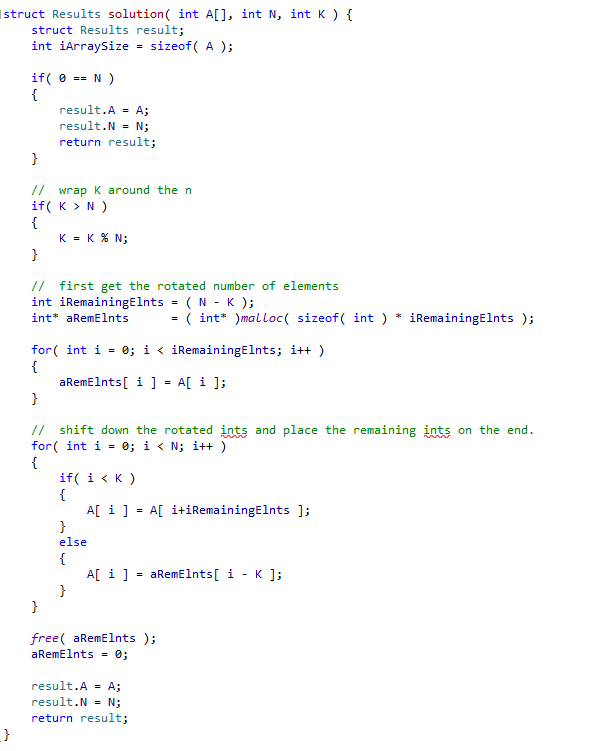You are to rotate an array of N integers K integers to the right. In this exercise time complexity is not scrutinised, only the correctness of your solution.

Language: C

Assumptions

N, K may range from 0 to 100. Therefore guard against empty arrays (N = 0)

Each element is within [-1000,1000], allow for negatives.

Solution

My solution rotates the array in-place, however a buffer is required in order to store the shifted ints at the front. So with A = [3, 8, 9, 7, 6] and K = 3 I store the 3 and the 8. I then shift the 9, 7 and 6 down (the K rotated ints) and put the remaining ints at the end.

You must use modulus (%) to wrap K around N if K > N. Obviously rotating by K = 6 is equivalent to rotating by K = 1.